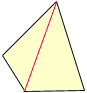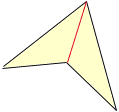Name: Zane Cram Who is asking: Other Level: All Question: I need the formula to calculate the area of an irregular sided rectangle. Each side has a different measurement or length. Hi Zane, I can draw the picture you are talking about BUT the name is wrong. The name is quadrilateral (four sides). RECTANGLE implies that the angles are right angles. There are fancy formule, but the essential method is DRAW A DIAGONAL.Find the area of the two triangles (assuming the diagonal runs through the interior of the object). Add up the areas of the two triangles. That is the area of the general quadrilateral. Walter Go to Math Central